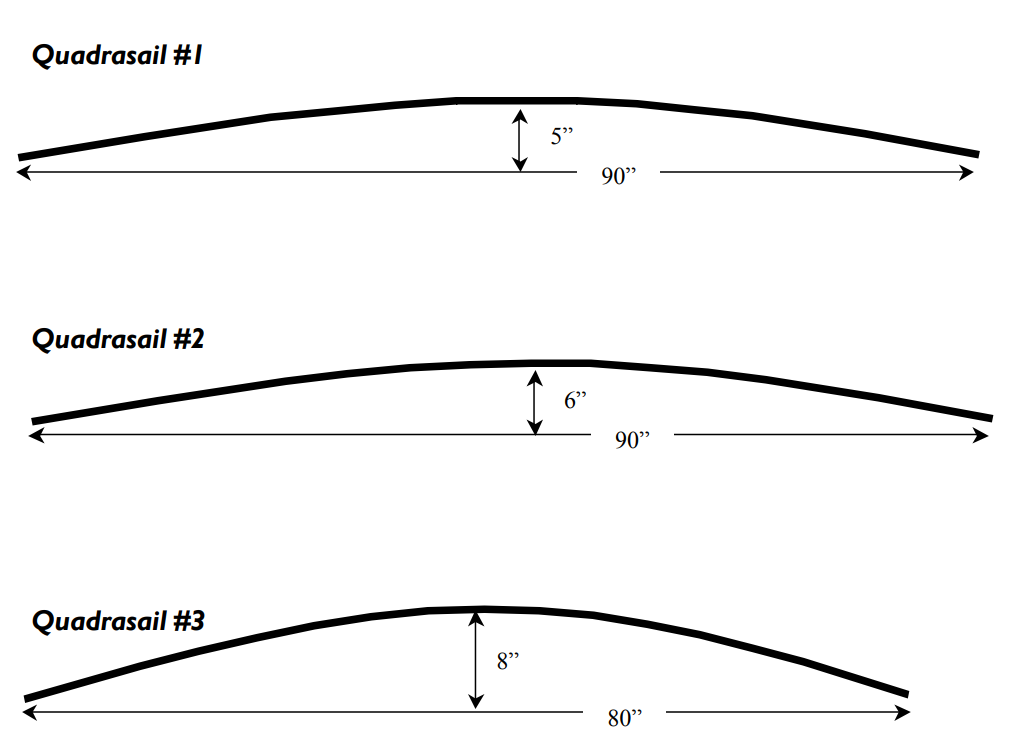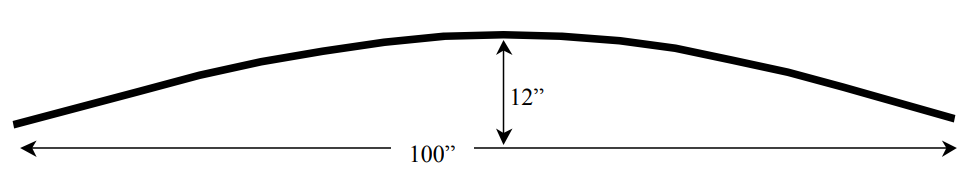Subject:
Mathematics

Common Core State Standard: A-CED.1, A-REI.10,
Concepts:

· Parabola

Knowledge and Skills:

· Can recognize a parabolic shape
· Can determine the equation of a quadratic function that contains three given points
· Can investigate how a family of curves can be produced by changing one parameter
· Can relate the coefficients of a quadratic function to their influence on the shape and position of the parabolic graph of that function

Lesson:

Procedure: This activity is best done with students working individually or in teams of two.

Distribute the handout and ensure that students understand the task.

1. 1: y = -x²/405 + 2x/9

2. y = -2x²/675 + 4x/15

3. y = -x²/200 + 2x/5 (all assuming that the left end of the mast is placed at the origin)

2. a = -12/10000, h = 100

### SurfSail Designs

From: Design Chief
For our new children’s “Quadrasail” design, we want to test several possible parabolic mast shapes:Task 1: Please determine the equation of each of the above curves, so that we can get these potential designs into the computer.

Task 2: The boss says we could produce a whole family of possible parabolic mast shapes, using this formula:

y = ax(x – h)   h = height of mast.

Let’s see if that’s right. Try these values for “a” for a mast which is 90” high, and show me what the resulting curves look like:

a = -4/(45*45)

a = -8/(45*45)

a = -6/(45*45)

Task #3: If we use the boss’s equation, what values of “a” and “h” should give the parabolic shape below?The boss is out testing sails today, so I’d like you to get this finished before the end of the day and on her desk.